Symbol
ProblemLearning Task 3. Complete the tables below and graph on the same coordinate plane. Then analyze the relationship between the graphs. 1. Given: $y=-2x2$ 2. Given: $y=-x2+4$ $Domnin$ Domain Range Range Opening of the Opening of the parabola parabola Vertex Vertex $Adsofsymmcty$ Axis of Symmetry $x-lntcrccpt$ x - intercept $y-intcrccpt$ $y-intcxccp$ 3. Given: $y=\left(x+1\right)2$ 4. Given: $y=2x^{2-4x+4}$ Domain $Dom0n$ Range Range $Opcningofthc$ Opening of the $paabola$ $paabola$ Vertex Vertex Axis of Symmetry Axis of Symmetry x- x - intercept intercept $y-intcrccp$ y- intercept 50 3. $-4$ $1$ $2$ $3|$ $5|$ 6 -7 $\bar{-6-5}$ $\bar{-4.3-2}$ -1 -2 -3 $+^{.5}|$ $y$ DARZON
7th-9th grade
Algebra
Search count: 122
SolutionQanda teacher - saishankar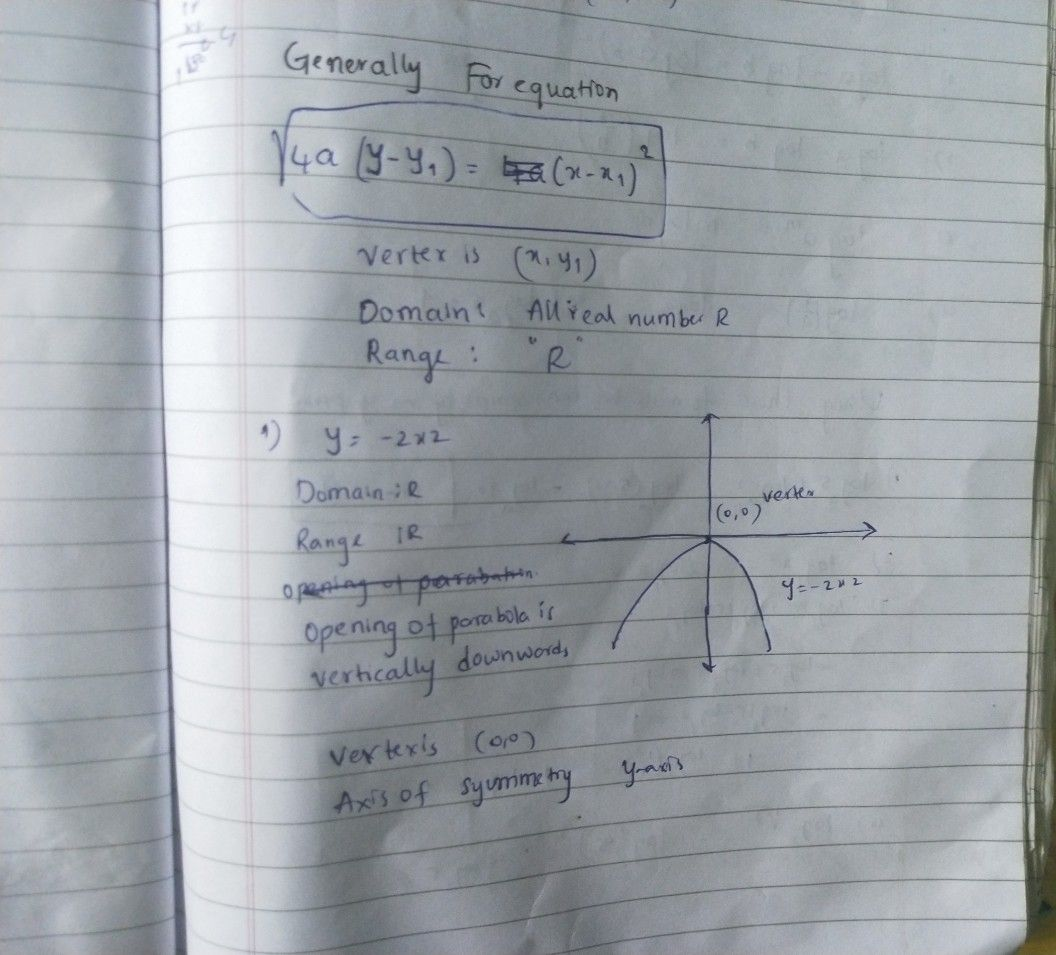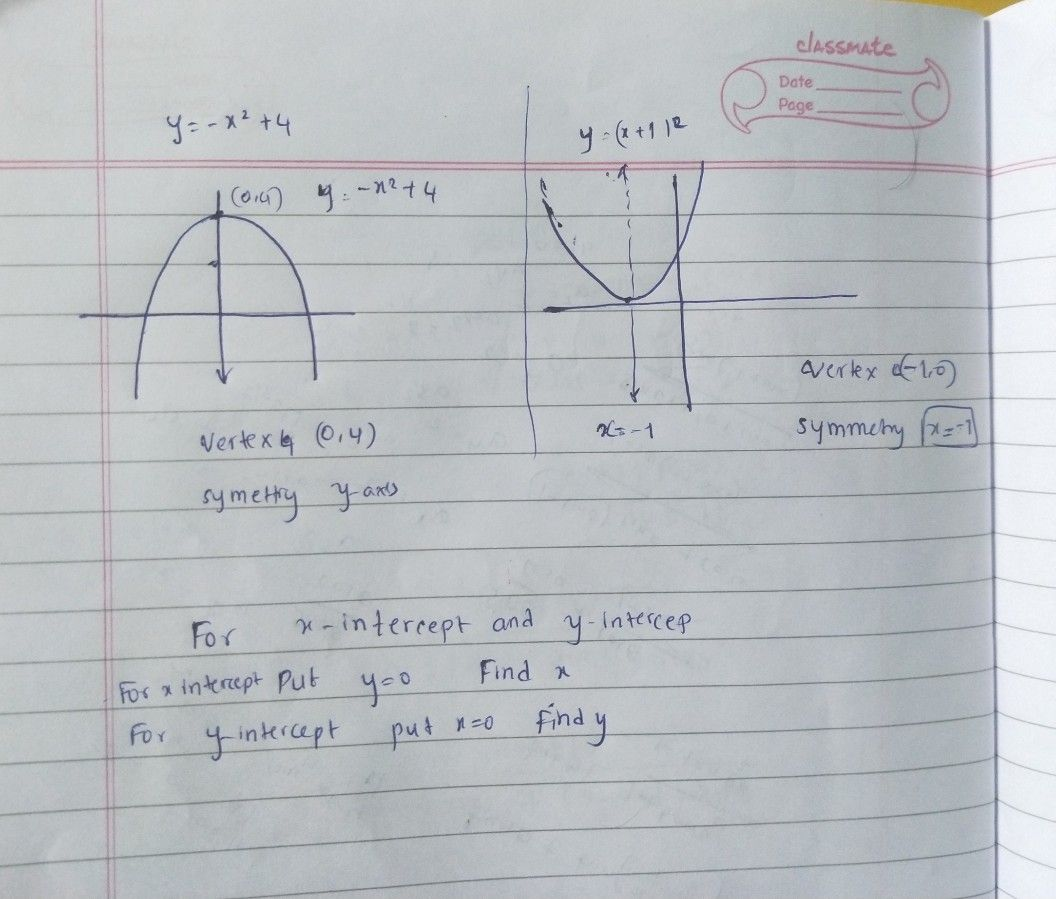thanks for using qandaStudent
can u plss put a number in the graph? so that i really know it?Qanda teacher - saishankar
okay just a minuteStudent
thank u sirQanda teacher - saishankar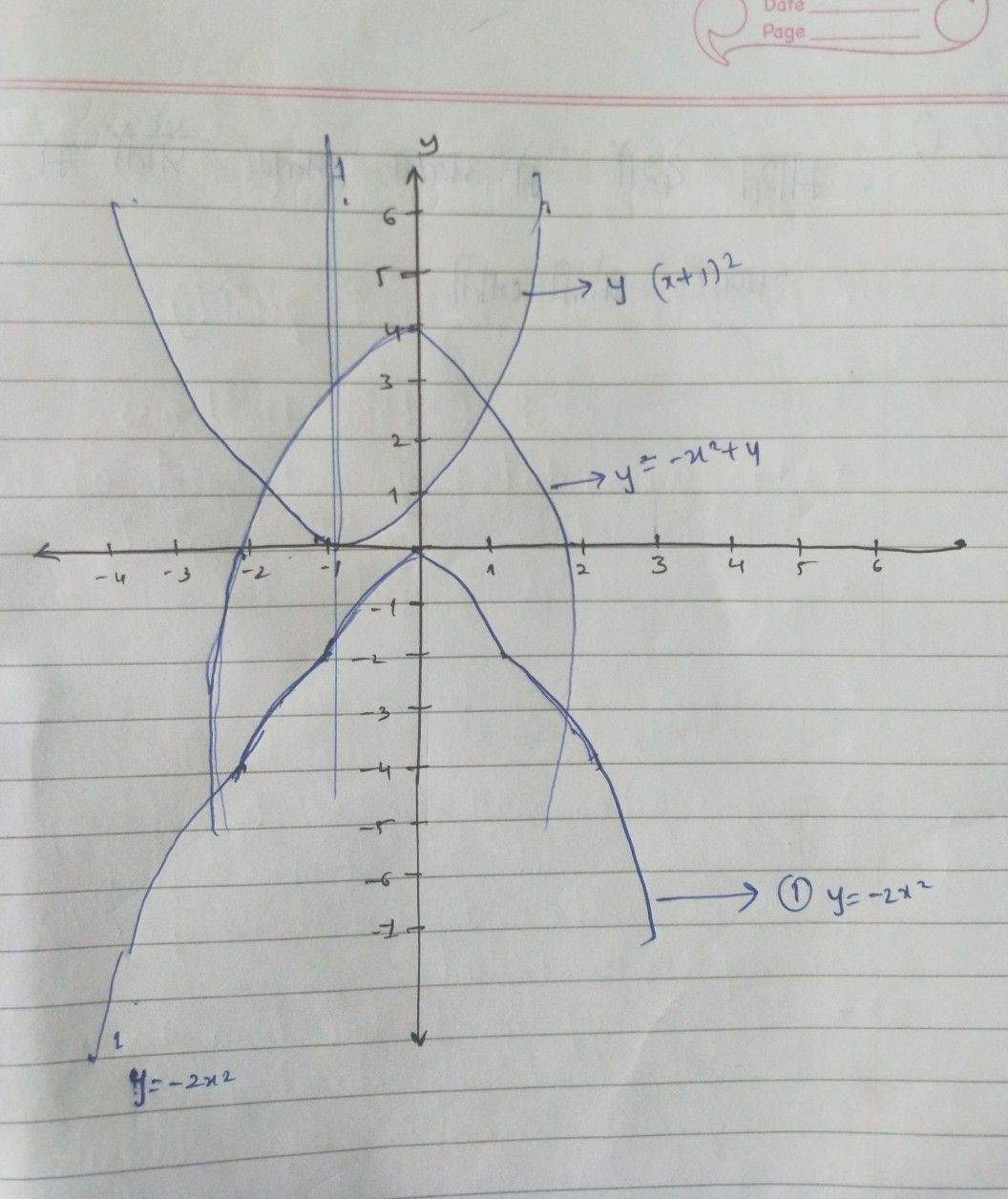Student
sir can u plss leave a circle in the number?
and wheres the number 4 sir?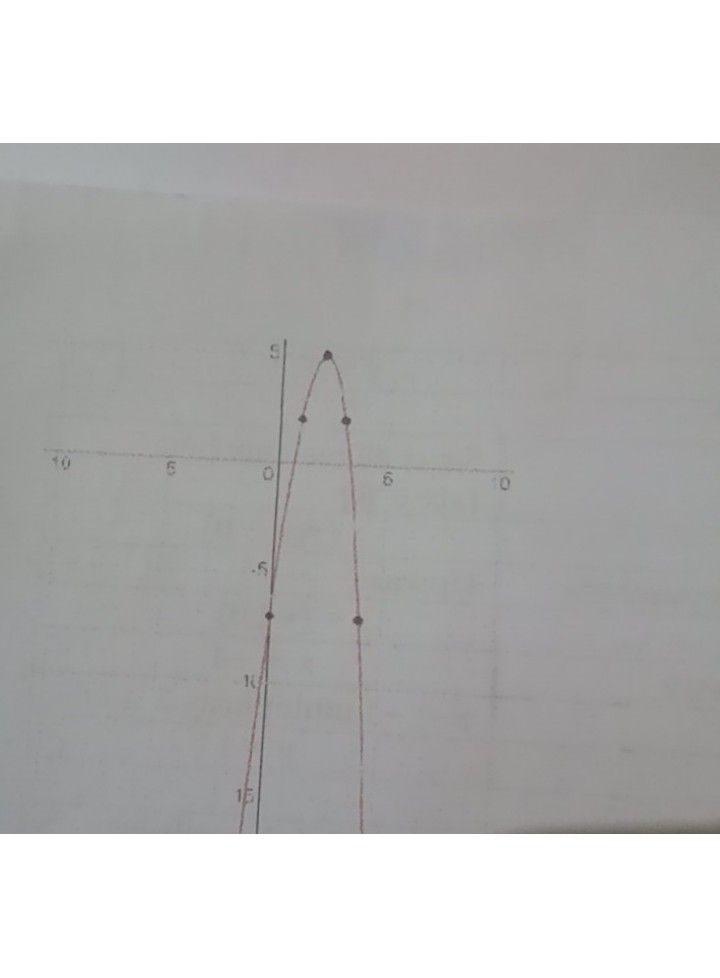just like these sir
that have a dotQanda teacher - saishankar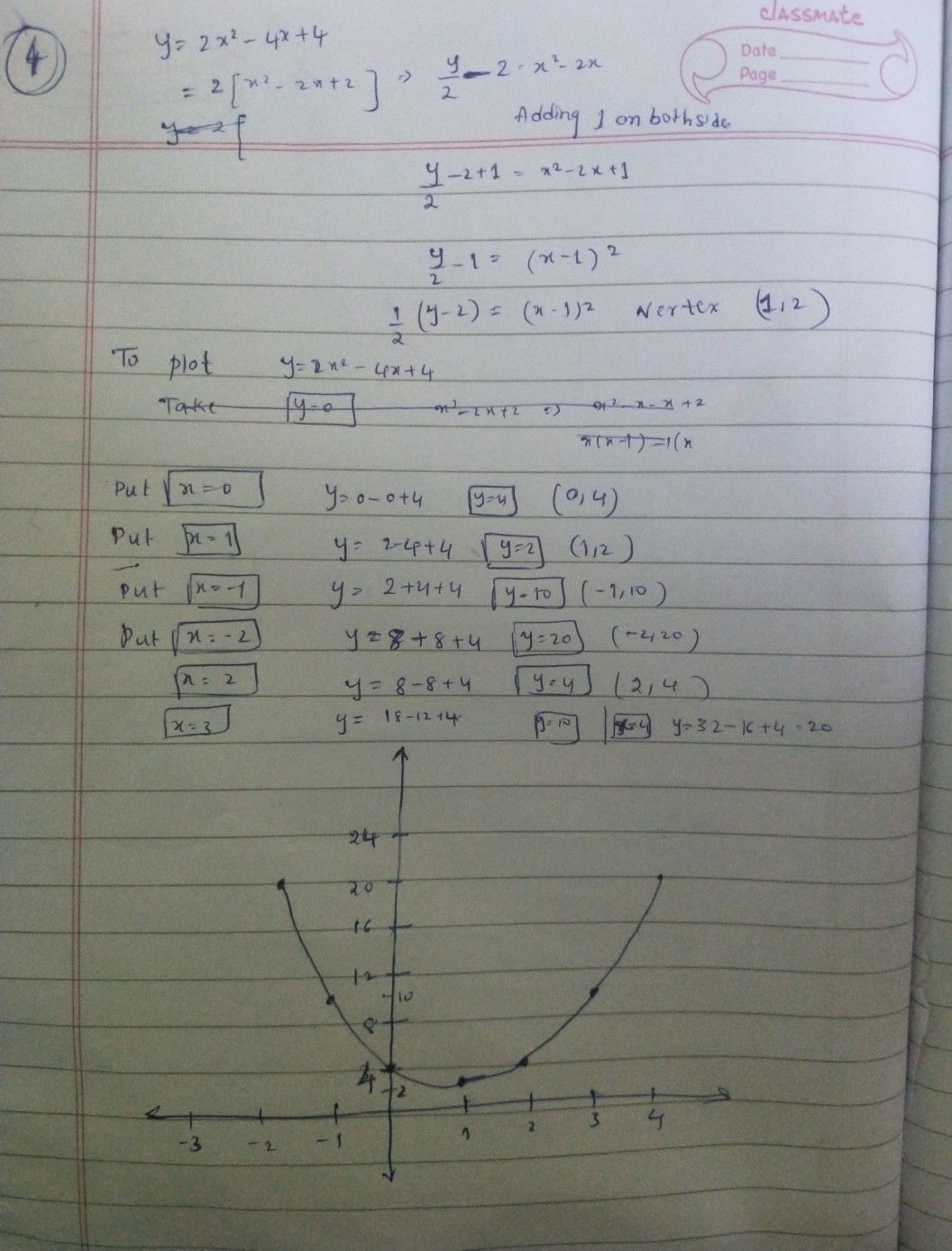Student
as well as the 1-3 you also put a dot sirQanda teacher - saishankar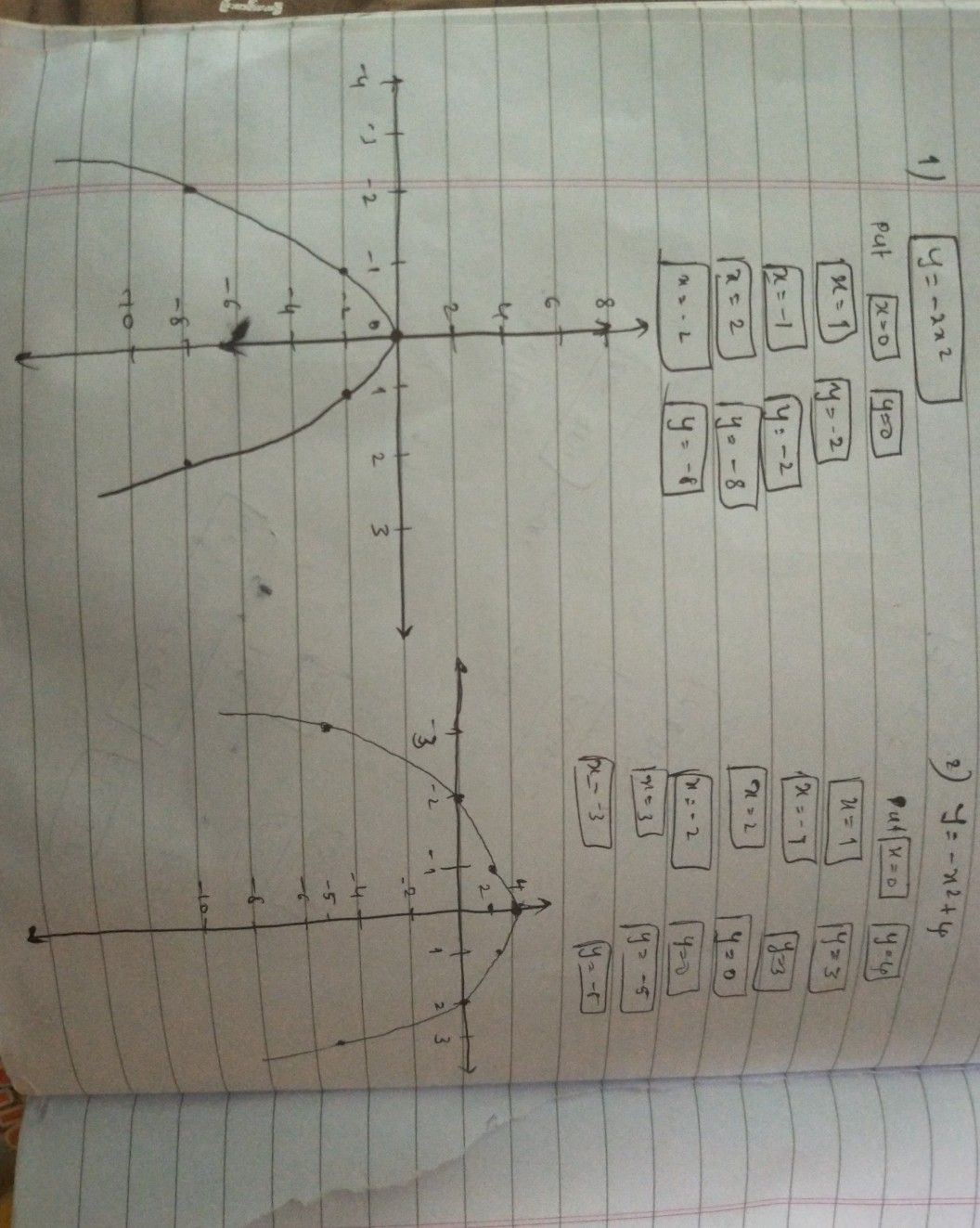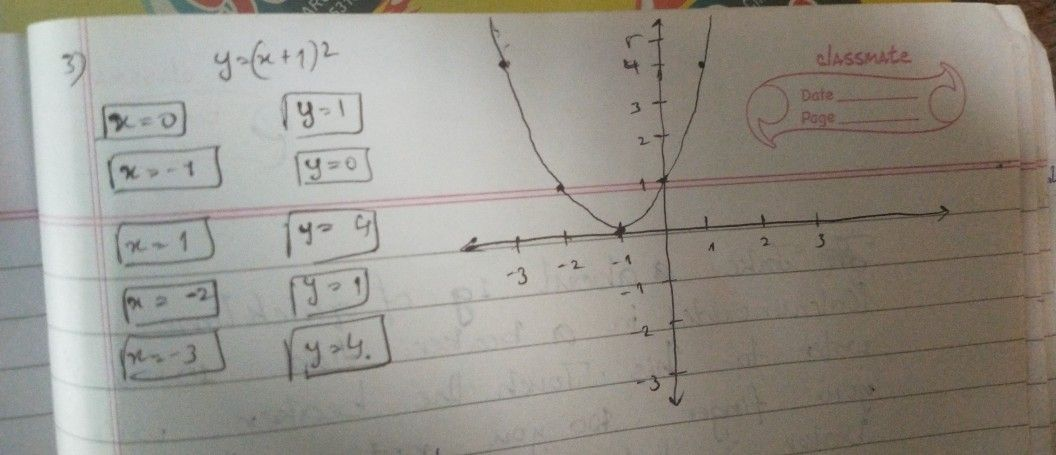Student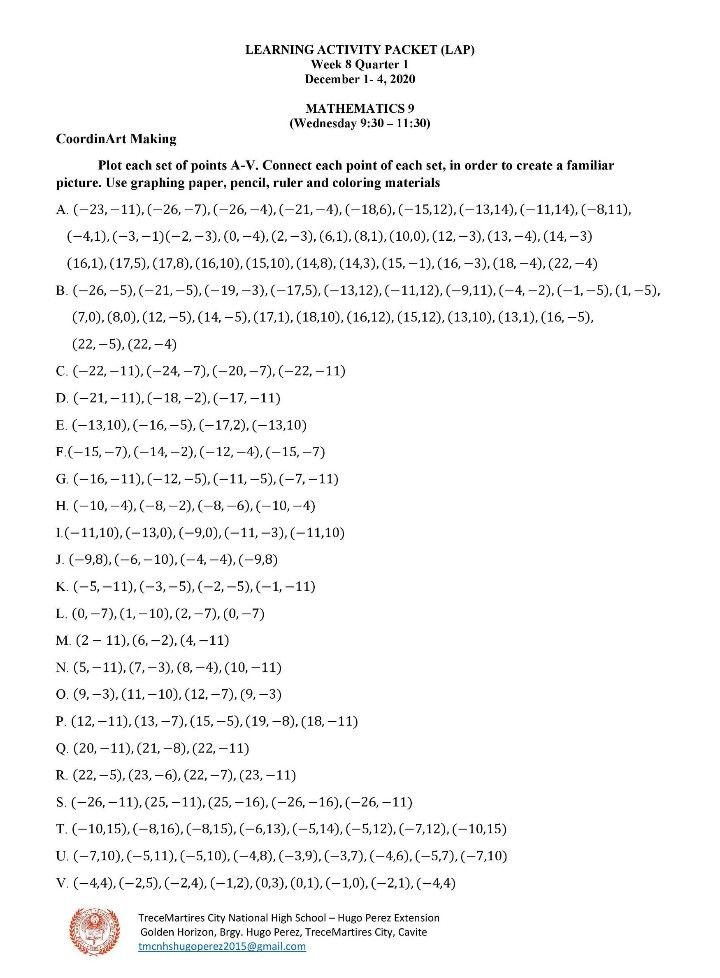sir can u plss answer this?Qanda teacher - saishankar
this is another question you need to ask separately as this is against qanda policy